# Bounce & Catch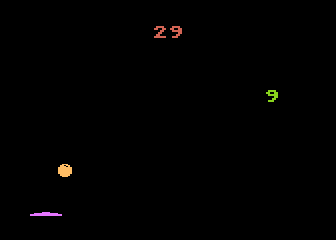This is a little game for the 2019 NOMAM's BASIC 10-liners Contest. This program fits in the PUR-80 category, and it was written using Atari BASIC for the 8-bits ATARI XL/XE.

## Description

Bounce the ball and catch all the numbers you can to score in 4 minutes. Triple points when hit from top.

## Instructions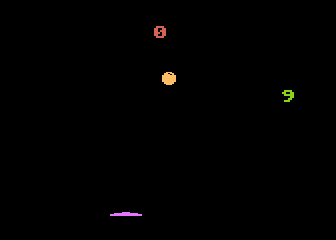A ball will appear in the middle of the playfield. Put the paddle bellow of it and avoid it to fall.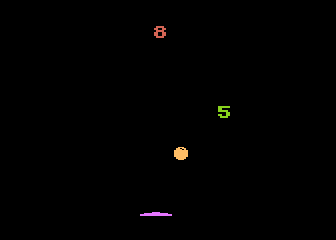You have to aim the ball against the green numbers. The angle of the ball depends on how you hit it with the paddle.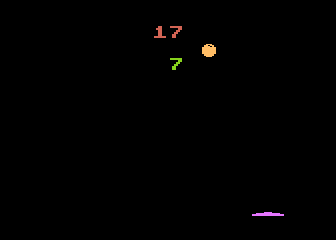Green numbers will decrease on each bounce. If you catch one, another will appear somewhere else. The score increases in the same amount that the catched number.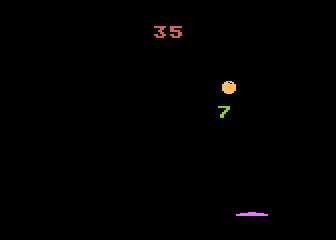If you catch a number from above, you will score the triple of points. The higher the number, a much better score.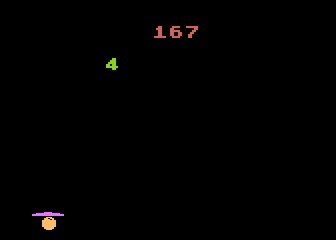The game is over when you miss the ball or when the time is over. Press the trigger to start another challenge.

## Development of the game

My kid was playing with a ping-pong paddle, continuously bouncing a ball trying to almost reach the ceiling, but without hitting it, and that gave me an idea for a game. While I was on the shower, I imagine some simple rules, but after some minutes, those changed to a whole new game. In my mind, there would be some numbers in the playfield and the bouncing ball must hit them to score, but there would also be some difficulties, because the paddle would be round and the bouncing direction would depend on the point of the paddle where the ball touches. Also, those numbers would decrease from time to time, and as the time goes, the lower would be the score. After one number is hit, it disappear and a new one appears in another location of the playfield. In addition, there would be a bonus when the number is hit from the top. At last, there would be only one live!

As I've just published UFO Attack!, another game that requires paddle controllers for the PUR-80 category, I wrote an Atari BASIC prototype for my new idea, just to test the speed of data manipulation using the strings trick, but in this case, managing Player/Missile graphics.

I wanted to perform a realistic bounce, so I used parabolic function to precalculate a table of heights for a small period of time. I wanted to use it in the tenliner, but that would use too much space in the listing to initialize an array or it would be too slow if it is evaluated on each game loop, so giving it as bytes in a constant string was much more simple. The result was quite good: I had a small sqare bouncing in the middle of the screen. Then, I added a simple way to move the square from side to side in a constant speed, and changed the sqare by a disk.

The next step was to add a paddle in the bottom of the screen. I choosed to use another player in quad width and shaped it a bit round, and allowed it to change the direction based on the difference of horizontal position between the paddle and the ball, and let the ball go away if the paddle was not bellow the ball.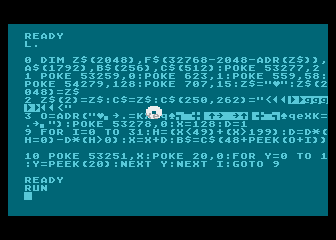Initial proof of concept of the bounces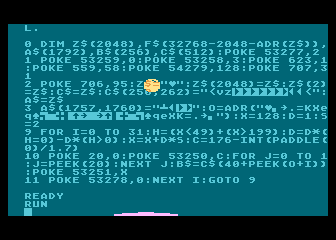Looking at the BASIC listing, I realized that all that P/M and string buffers preparation took many 80 bytes lines, and there was not so much space to code the game rules. I sat in front of the screen an saw what things could be changed to save space without loosing what has been done.

After some optimizations, I decided to use only one target at a time, because there was no room to initialize an array and manage it. After some fine tunning, I had a working and playable game.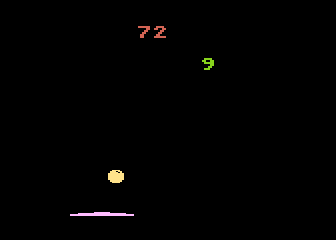First version of the game

The program was using less than 800 bytes in total, but required more than 10 lines because of the conditional code. Loops were implemented with FOR-NEXT statements, but I had no way to implement an IF-THEN-ELSE structure without the use of GOTO statement.

As some blocks of instructions has to be conditionaly performed as a whole, I had two alternatives: all that block must fit in a single line starting with an IF-THEN statement, or use ON-GOTO to jump over the block if more than one line or less than one line was required. After some optimizations and code simplification, I managed to use both techniques, as I identified 4 statements that should be the destination of a conditional jump, including loops. To fit all instructions in the available space, I had to allow multiple executions of some statements by leaving them inside the loops instead of outside, with a penalty in the speed, but fortunately not in the game loop.

More code simplification allowed me to add a bonus score and better sound effects, but during my tests, the game became boring because it was easy even with only one life, as the player could keep bouncing the ball forever trying to score. A simple way to add extra difficulty was to reduce the size of the paddle from quad to double. Many test games later, I also add another end of game condition, but I had to reacomodate all the initialization code again to be able to set up an extra variable for the bouncing counter. As all bounces lasts the same amount of time, to finish the game after, say, 4 minutes, I just had to count 62 bounces.

Finally, I decided to do more fine tunning, and change the ball to be only 7 pixels wide. This way, the number of posible collisions of the ball and the paddle are an even number, and then to have the same number of bounces to the left than to the right, and no bounces exactly in the middle of the paddle.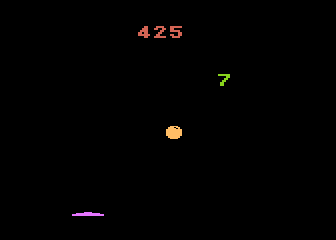A narrower ball with time limit

Get the BOUNCE.ATR file and set it as drive 1 in a real Atari with paddle controllers in port 1. Turn on the computer with BASIC enabled (without pressing OPTION key) and the game should start after loading.

Using a mouse as a paddle: even when mouse does not replace the experience of a real paddle controller, it is possible to play this game in emulators using the mouse to control the paddle.

TurboBasic XL hack! To play this game using TurboBasic XL, you must change memory address in the assignment of Q variable at line 1: 48792 instead of 40600. The game will be run faster, but it will also last less than with Atari BASIC.

## The code

The abbreviated BASIC code of Bounce & Catch is the following: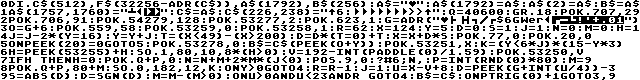The full and expanded BASIC listing is:

 ```0 ``` LINE 0: Initialize strings. ```dim c\$(512),f\$(32256-adr(c\$)),a\$(1792),b\$(256) ``` Reserves memory for game elements data, forcing A\$ to be at page 128 (\$80) matching PMBASE. ```a\$="{binary zero}" a\$(1792)=a\$ a\$(2)=a\$ b\$=a\$ ``` Clears P/M area. ```1 ``` LINE 1: Initialize graphics mode. ```a\$(1757,1760)="{binary string}" ``` Puts the paddle in the bottom of P3 at P/M area. ```c\$=a\$ c\$(226,238)="{binary string}" ``` Sets up a ball buffer. ```q=40600 ``` Screen memory to place targets (SAVMSC is at 40560) (TurboBASIC hack: use 48792). ```graphics 18 ``` Set screen to graphics mode 2 (ANTIC 7) without text window. ```poke 707,29 ``` Colors for the ball. ```2 ``` LINE 2:Sets up P/M graphics. ```poke 706,91 ``` Colors for paddle. ```poke 54279,128 ``` Sets PMBASE. ```poke 53277,2 ``` GRACTL=2: Players only, no missiles. ```poke 623,1 ``` GPRIOR=1: Players over playfield. ```g=adr("{binary string}") ``` Bouncing directions table (6 bytes) and altitude table (17 bytes). ```3 ``` LINE 3: Initializes game variables. ```o=g+6 ``` Sets memory address of altitude table. ```poke 559,58 ``` SDMCTL=2+32+8+16: One line resolution players only (no missiles) in standard playfield. ```poke 53259,0 poke 53258,1 ``` Sets P3 in normal width, P2 in double width. ```r=62 ``` Sets max number of bounces (62 bounces ~ 4 minutes). ```x=124 y=5 ``` Initial position of the ball. ```d=0 s=1 j=1 ``` Initial speed and direction. ```n=0 ``` Resets score. ```m=0 ``` Sets current target as 0 points. ```h=1 ``` Forces a fake hit to add a target at the game start. ```4 ``` LINE 4: GAME LOOP ```j=j-2*(y=16) y=y+j t=(x<49)-(x>200) d=d*(t=0)+t x=x+d*s ``` Calculates next position of the ball. ```poke 77,0 ``` Resets ATRACT counter. ```poke 20,0 ``` Resets timer. ```5 ``` LINE 5: Sinchronizes to VBLANK ```on peek(20)=0 goto 5 ``` Forces a VBLANK to detect a collision. ```poke 53278,0 ``` Cleans the collision registers. ```b\$=c\$(peek(o+y)) poke 53251,x ``` Puts the ball in its new position. ```k=(y<6*j)*(15-y*3) ``` Has the ball just bounce? Calculates the volume of the bouncing sound: - Plays sound only if y<6 and j>0 - The volume depends on the height ```6 ``` LINE 6: Detects for collisions and sets up sound. ```h=peek(53255)+h ``` Detects a collision between tha ball and the target. ```sound 1,80,10,8*(h>0) ``` Plays a sound when a collision has been detected (and at the start of the game). ```v=192-int(paddle(0)/1.59) poke 53250,v ``` Draws the current position of the paddle. ```7 ``` LINE 7: Sets up a new target. ```if h then h=0 ``` If the target was hit, clears the hit flag, ``` poke q+p,0 ``` removes the target, ``` n=n+m+2*m*(j<0) ``` increases the score with bonus if ball is falling, ``` position 9,0 ?#6;n, ``` displays the new score, ``` p=int(rnd(0)*80) m=9 ``` and creates a new target. ```8 ``` LINE 8: Loops unless the ball is at the bottom. ```poke q+p,80+m ``` Displays the target with its current value. ```sound 0,182,12,k ``` Performs the bouncing sound. ```on y>0 goto 4 ``` Loops if the ball is not at the bottom level. ```r=r-1 ``` Decreases number of remaining bounces. ```j=1 ``` Going up again. ```u=x-v+8 d=peek(g+int(u/4))-3 ``` Checks if the paddle is under the ball. An index out of range is possible and it will give garbage at PEEK, but it is discarded later. ```9 ``` LINE 9: Loops unless the game is over. ```s=abs(d) d=sgn(d) ``` Calculates the new deltas for the ball horizontal movement. ```m=m-(m>0) ``` Decreases the target value. ```on u>0 and u<23 and r goto 4 ``` Loops if the paddle was under the ball (and the index was within the range), and time is not over. ```b\$=c\$ ``` Failed to hit the ball or time limit. Displays the ball under the paddle. ```on ptrig(0)+1 goto 3,9 ``` Waits for the trigger to restart the game.